Best for live in-class or video conferencing lessonsStart teacher-led lesson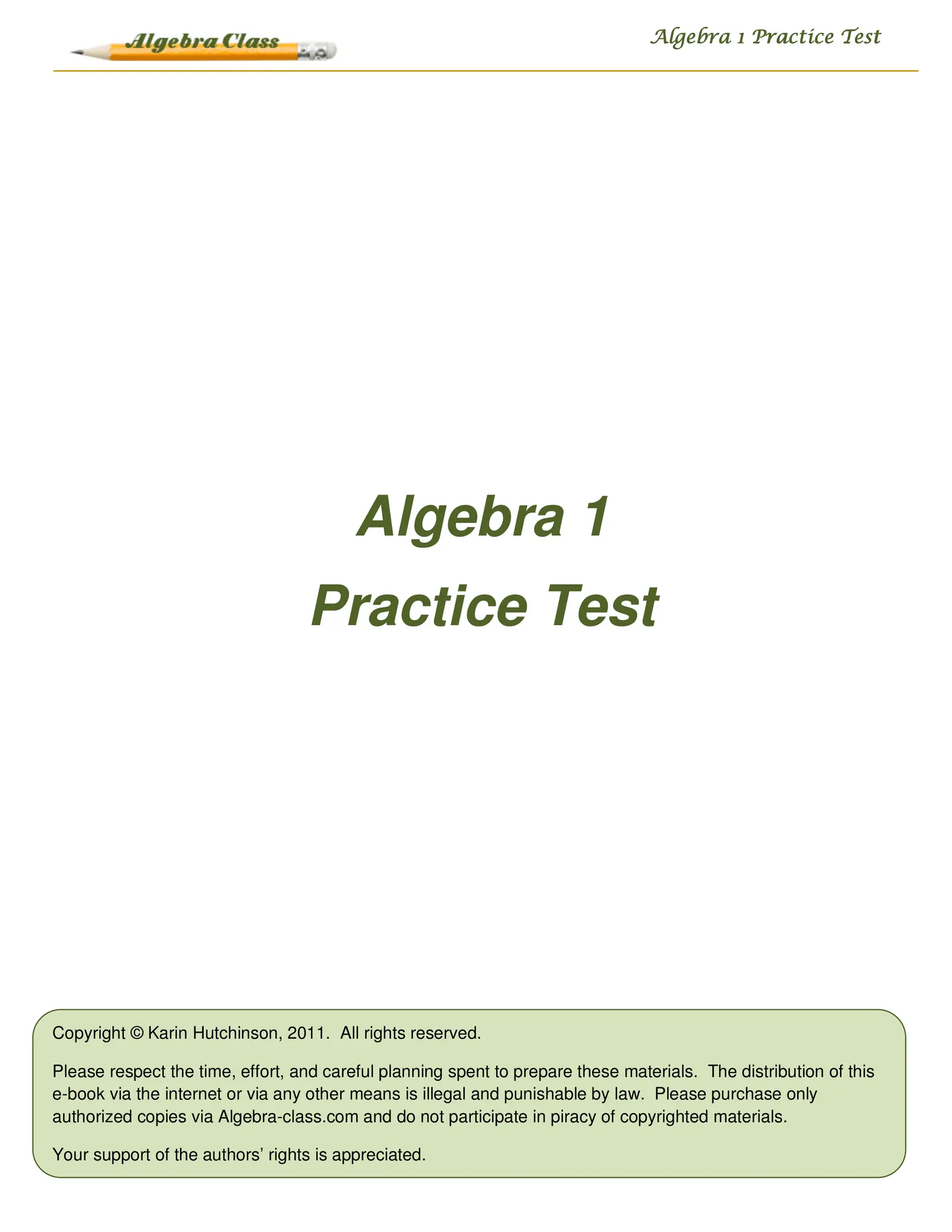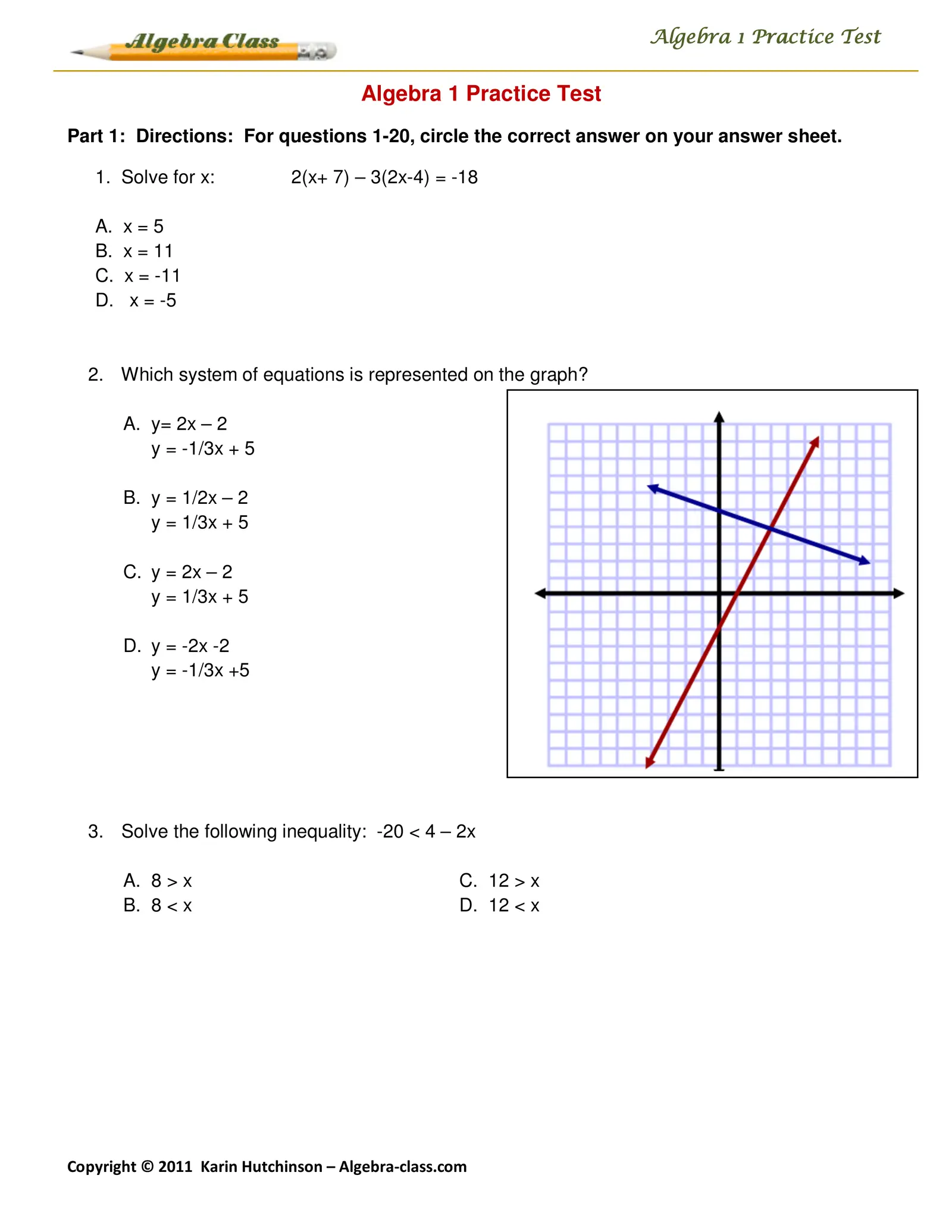Algebra 1 Practice Test Algebra 1 Practice Test Part 1: Directions: For questions 1-20, circle the correct answer on your answer sheet. 1. Solve for x: 2(x+ 7) – 3(2x-4) = -18 A. x=5 B. x = 11 C. x = -11 D. x = -5 2. Which system of equations is represented on the graph? A. y= 2x – 2 y = -1/3x + 5 B. y = 1/2x – 2 y = 1/3x + 5 C. y = 2x – 2 y = 1/3x + 5 D. y = -2x -2 y = -1/3x +5 3. Solve the following inequality: -20 < 4 – 2x A. 8 > x C. 12 > x B. 8 < x D. 12 < x Copyright © 2011 Karin Hutchinson – Algebra-class.com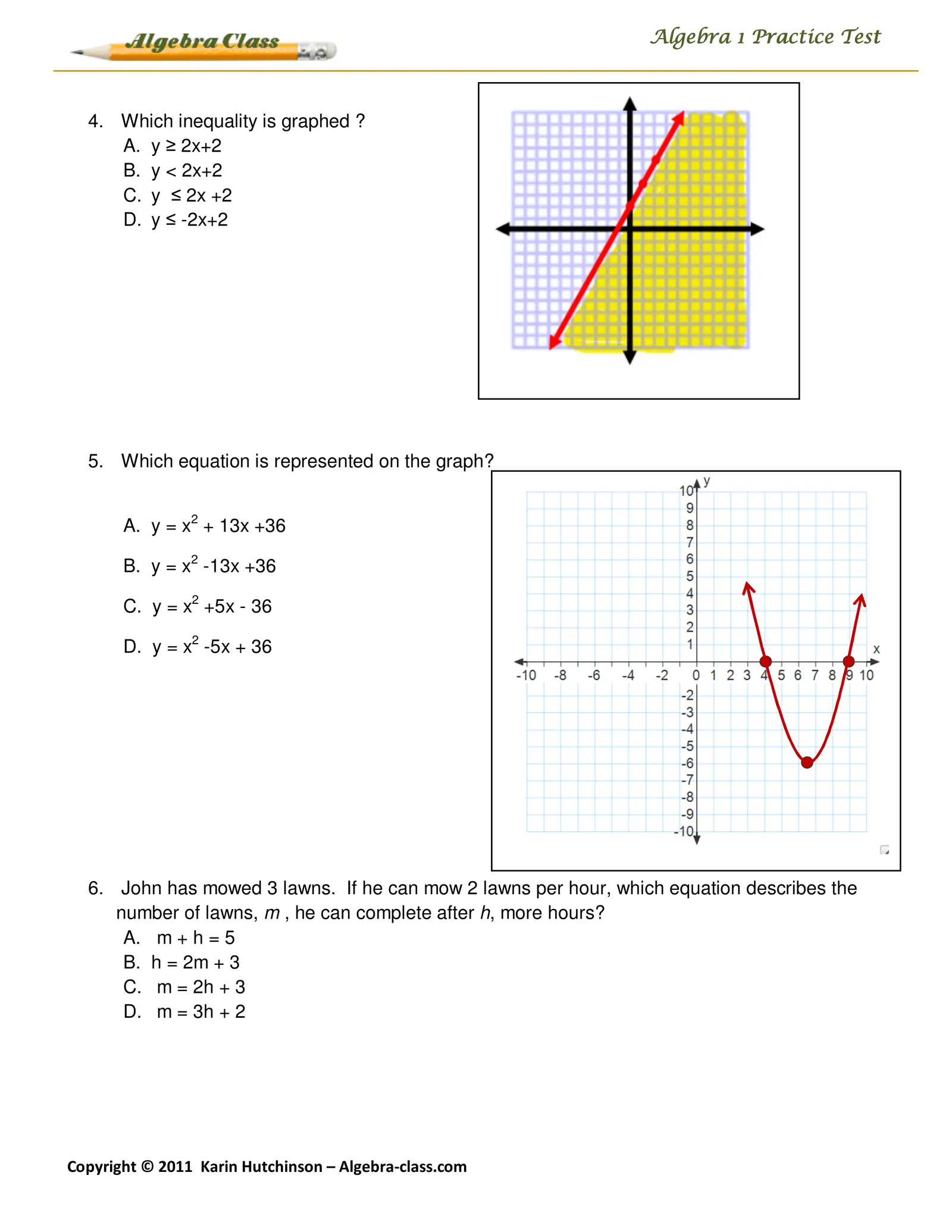Algebra 1 Practice Test 4. Which inequality is graphed ? A. y ≥ 2x+2 B. y < 2x+2 C. y ≤ 2x +2 D. y ≤ -2x+2 5. Which equation is represented on the graph? A. y = x2 + 13x +36 B. y = x2 -13x +36 C. y = x2 +5x - 36 D. y = x2 -5x + 36 6. John has mowed 3 lawns. If he can mow 2 lawns per hour, which equation describes the number of lawns, m , he can complete after h, more hours? A. m + h = 5 B. h = 2m + 3 C. m = 2h + 3 D. m = 3h + 2 Copyright © 2011 Karin Hutchinson – Algebra-class.com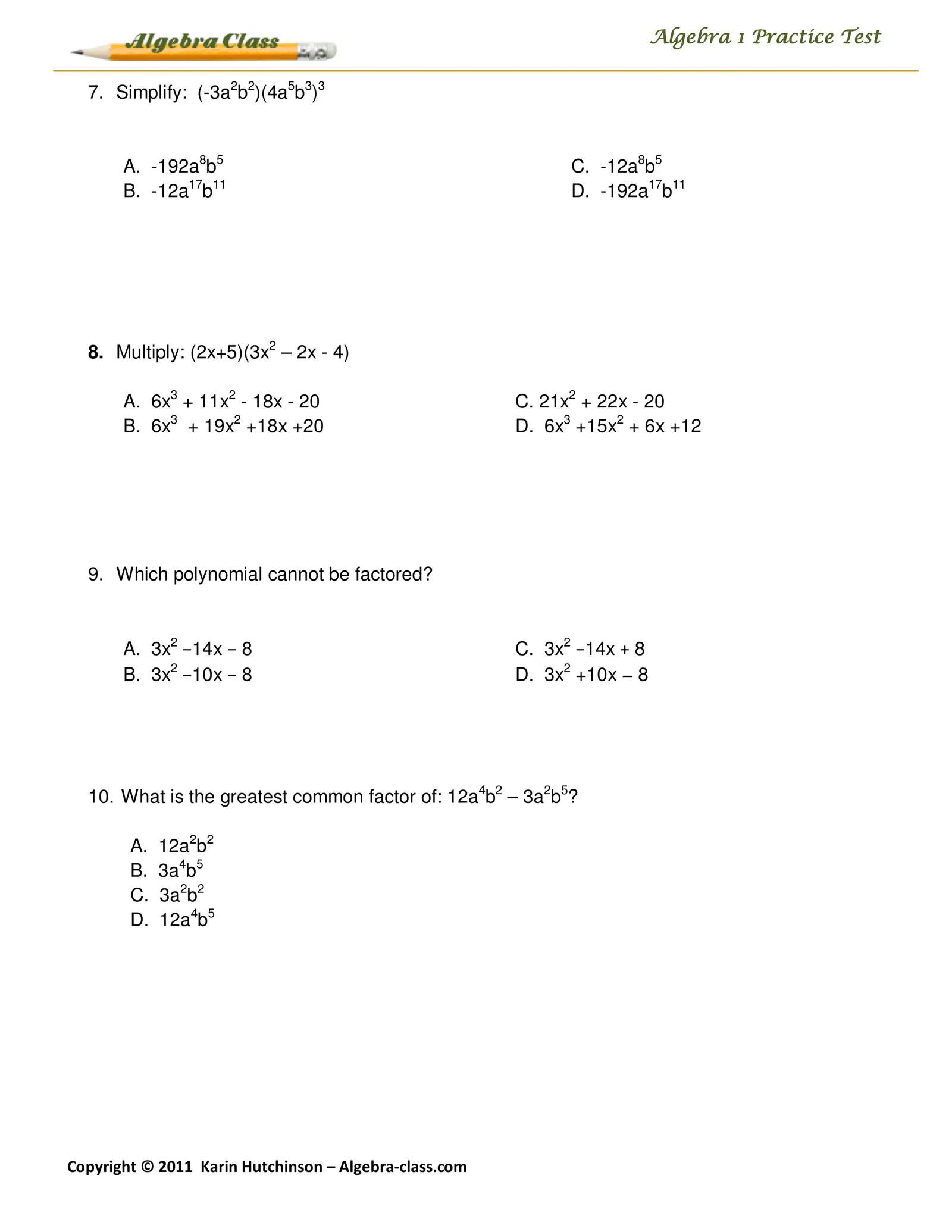Algebra 1 Practice Test 7. Simplify: (-3a2b2)(4a5b3)3 A. -192a8b5 C. -12a8b5 B. -12a17b11 D. -192a17b11 8. Multiply: (2x+5)(3x2 – 2x - 4) A. 6x3 + 11x2 - 18x - 20 C. 21x2 + 22x - 20 B. 6x3 + 19x2 +18x +20 D. 6x3 +15x2 + 6x +12 9. Which polynomial cannot be factored? A. 3x2 −14x − 8 C. 3x2 −14x + 8 B. 3x2 −10x − 8 D. 3x2 +10x − 8 10. What is the greatest common factor of: 12a4b2 – 3a2b5? A. 12a2b2 B. 3a4b5 C. 3a2b2 D. 12a4b5 Copyright © 2011 Karin Hutchinson – Algebra-class.com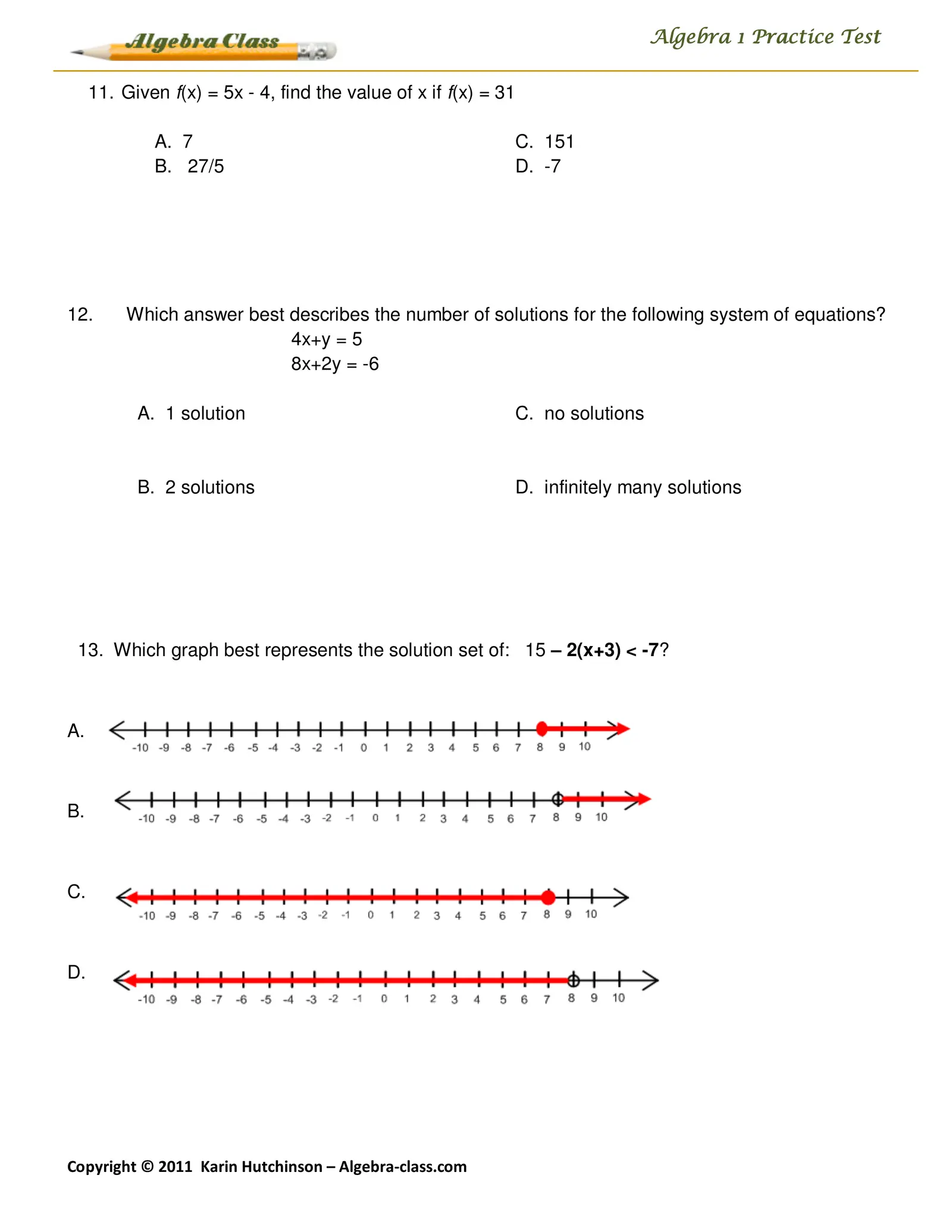Algebra 1 Practice Test 11. Given f(x) = 5x - 4, find the value of x if f(x) = 31 A. 7 C. 151 B. 27/5 D. -7 12. Which answer best describes the number of solutions for the following system of equations? 4x+y = 5 8x+2y = -6 A. 1 solution C. no solutions B. 2 solutions D. infinitely many solutions 13. Which graph best represents the solution set of: 15 – 2(x+3) < -7? A. B. C. D. Copyright © 2011 Karin Hutchinson – Algebra-class.com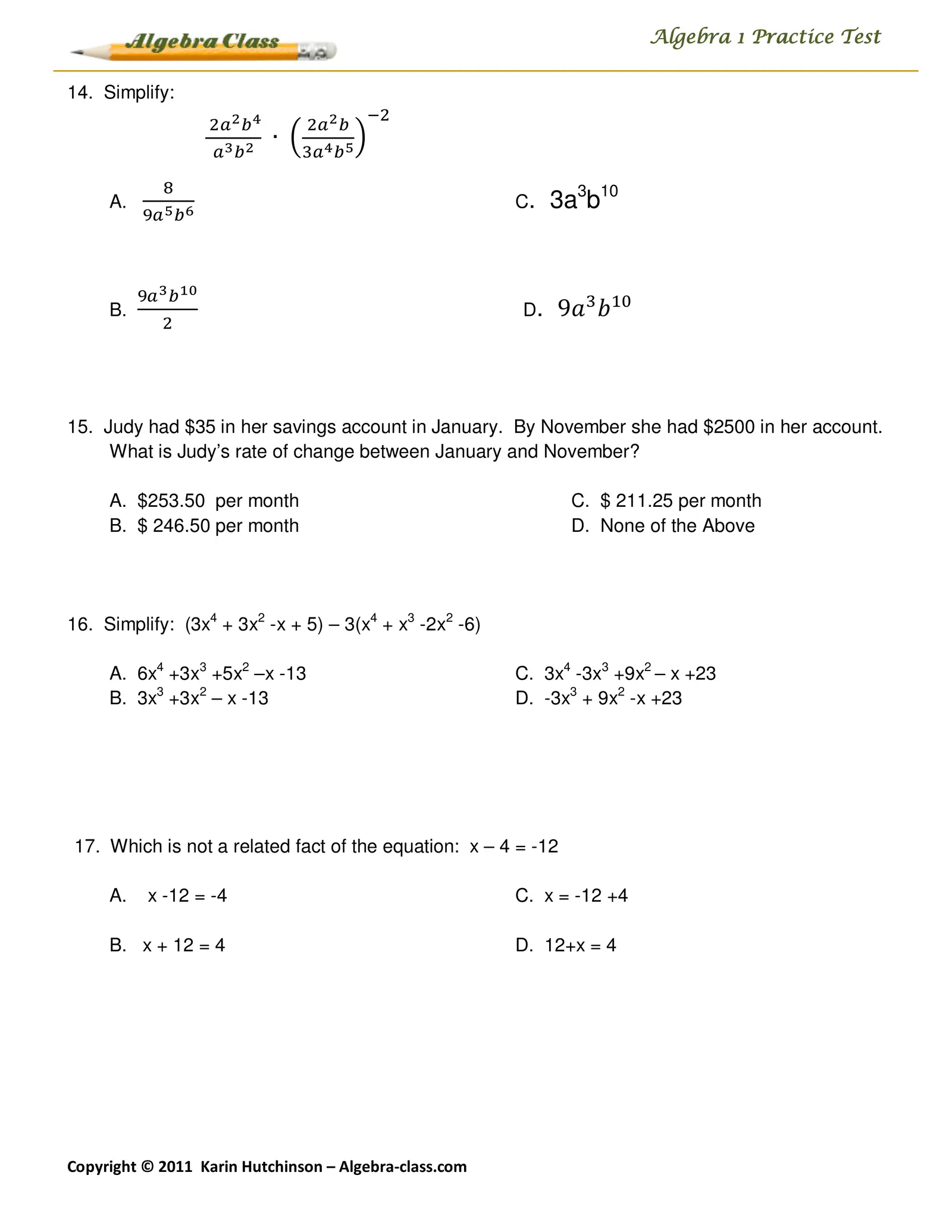Algebra 1 Practice Test 14. Simplify: ିଶ ଶ௔మ ௕ర ଶ௔మ ௕ ∙ ቀ ቁ ௔య ௕మ ଷ௔ర ௕ఱ ଼ A. C. 3a3b10 ଽ௔ఱ ௕ల ଽ௔య ௕భబ B. D. 9ܽଷ ܾଵ଴ ଶ 15. Judy had \$35 in her savings account in January. By November she had \$2500 in her account. What is Judy’s rate of change between January and November? A. \$253.50 per month C. \$ 211.25 per month B. \$ 246.50 per month D. None of the Above 16. Simplify: (3x4 + 3x2 -x + 5) – 3(x4 + x3 -2x2 -6) A. 6x4 +3x3 +5x2 –x -13 C. 3x4 -3x3 +9x2 – x +23 B. 3x3 +3x2 – x -13 D. -3x3 + 9x2 -x +23 17. Which is not a related fact of the equation: x – 4 = -12 A. x -12 = -4 C. x = -12 +4 B. x + 12 = 4 D. 12+x = 4 Copyright © 2011 Karin Hutchinson – Algebra-class.com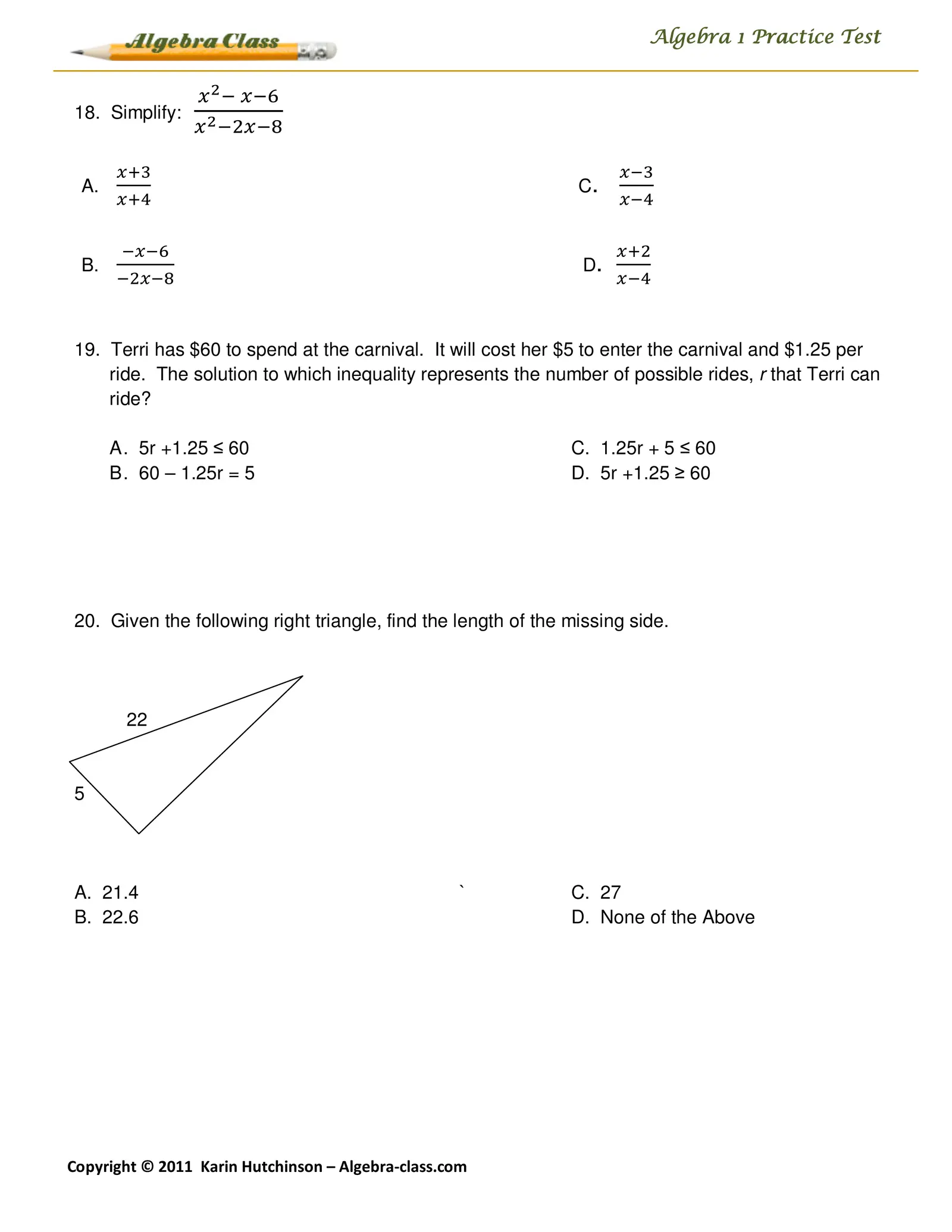Algebra 1 Practice Test ௫ మ ି ௫ି଺ 18. Simplify: ௫ మ ିଶ௫ି଼ ௫ାଷ ௫ିଷ A. C. ௫ାସ ௫ିସ ି௫ି଺ ௫ାଶ B. D. ିଶ௫ି଼ ௫ିସ 19. Terri has \$60 to spend at the carnival. It will cost her \$5 to enter the carnival and \$1.25 per ride. The solution to which inequality represents the number of possible rides, r that Terri can ride? A . 5r +1.25 ≤ 60 C. 1.25r + 5 ≤ 60 B . 60 – 1.25r = 5 D. 5r +1.25 ≥ 60 20. Given the following right triangle, find the length of the missing side. 22 5 A. 21.4 ` C. 27 B. 22.6 D. None of the Above Copyright © 2011 Karin Hutchinson – Algebra-class.com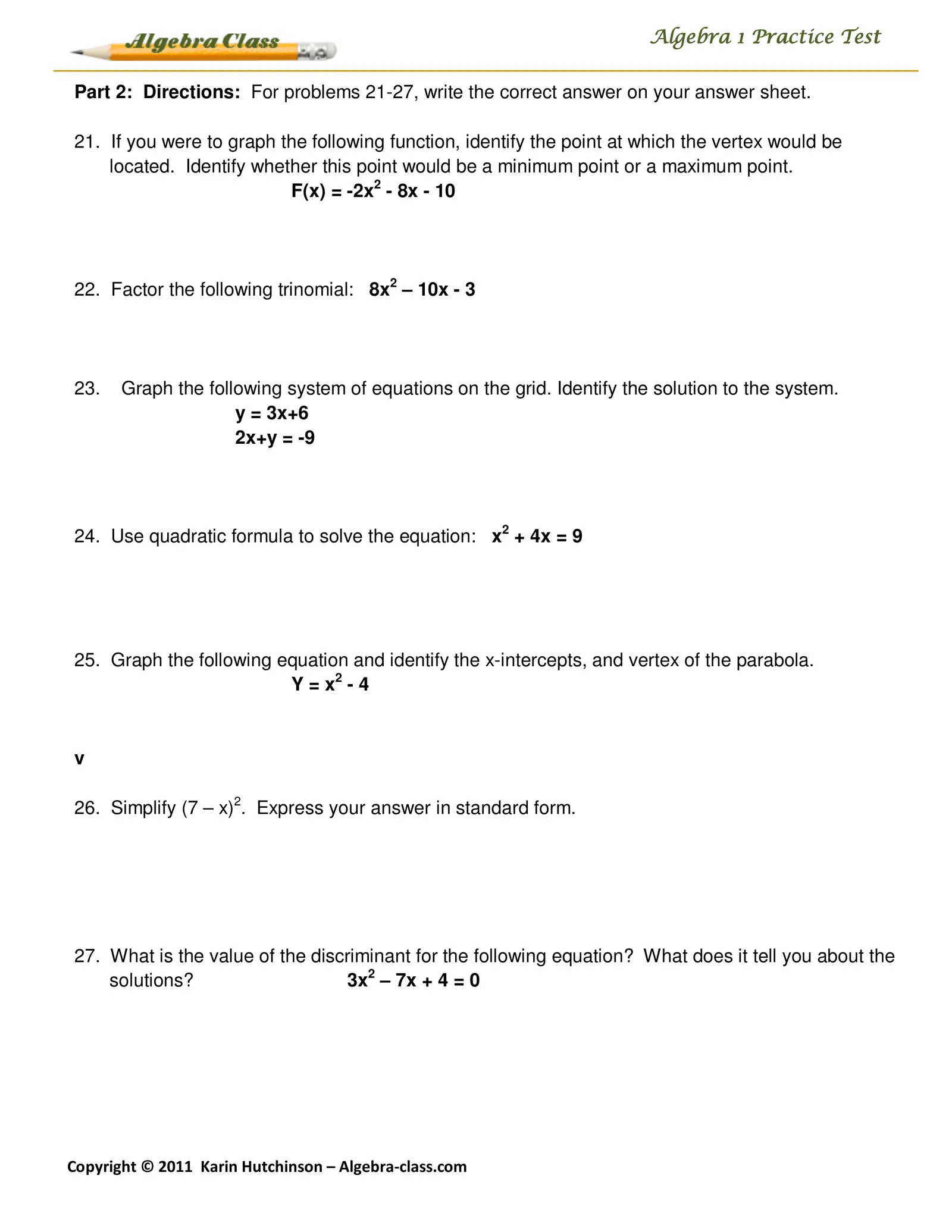Algebra 1 Practice Test Part 2: Directions: For problems 21-27, write the correct answer on your answer sheet. 21. If you were to graph the following function, identify the point at which the vertex would be located. Identify whether this point would be a minimum point or a maximum point. F(x) = -2x2 - 8x - 10 22. Factor the following trinomial: 8x2 – 10x - 3 23. Graph the following system of equations on the grid. Identify the solution to the system. y = 3x+6 2x+y = -9 24. Use quadratic formula to solve the equation: x2 + 4x = 9 25. Graph the following equation and identify the x-intercepts, and vertex of the parabola. Y = x2 - 4 v 26. Simplify (7 – x)2. Express your answer in standard form. 27. What is the value of the discriminant for the following equation? What does it tell you about the solutions? 3x2 – 7x + 4 = 0 Copyright © 2011 Karin Hutchinson – Algebra-class.com

X
The discriminant is
There are
rational solutions.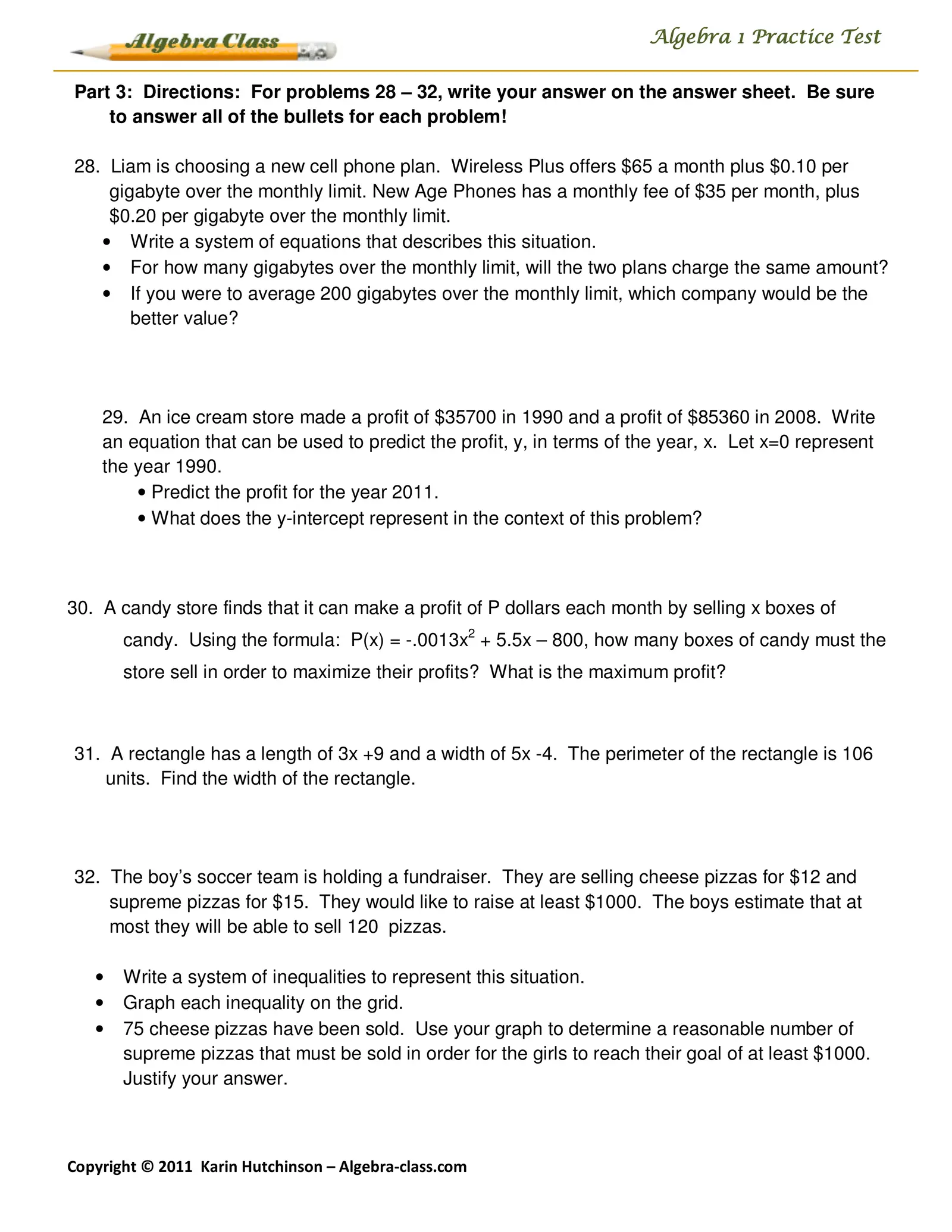Algebra 1 Practice Test Part 3: Directions: For problems 28 – 32, write your answer on the answer sheet. Be sure to answer all of the bullets for each problem! 28. Liam is choosing a new cell phone plan. Wireless Plus offers \$65 a month plus \$0.10 per gigabyte over the monthly limit. New Age Phones has a monthly fee of \$35 per month, plus \$0.20 per gigabyte over the monthly limit. • Write a system of equations that describes this situation. • For how many gigabytes over the monthly limit, will the two plans charge the same amount? • If you were to average 200 gigabytes over the monthly limit, which company would be the better value? 29. An ice cream store made a profit of \$35700 in 1990 and a profit of \$85360 in 2008. Write an equation that can be used to predict the profit, y, in terms of the year, x. Let x=0 represent the year 1990. • Predict the profit for the year 2011. • What does the y-intercept represent in the context of this problem? 30. A candy store finds that it can make a profit of P dollars each month by selling x boxes of candy. Using the formula: P(x) = -.0013x2 + 5.5x – 800, how many boxes of candy must the store sell in order to maximize their profits? What is the maximum profit? 31. A rectangle has a length of 3x +9 and a width of 5x -4. The perimeter of the rectangle is 106 units. Find the width of the rectangle. 32. The boy’s soccer team is holding a fundraiser. They are selling cheese pizzas for \$12 and supreme pizzas for \$15. They would like to raise at least \$1000. The boys estimate that at most they will be able to sell 120 pizzas. • Write a system of inequalities to represent this situation. • Graph each inequality on the grid. • 75 cheese pizzas have been sold. Use your graph to determine a reasonable number of supreme pizzas that must be sold in order for the girls to reach their goal of at least \$1000. Justify your answer. Copyright © 2011 Karin Hutchinson – Algebra-class.com

Wireless Plus:
New Age Phone:
The candy store must sell
boxes of candy in order to maximize its profit.
The maximum profit would be \$
The width of the rectangle is
units
Let x = number of cheese pizzas. Let y = number of supreme pizzas
If 75 cheese pizzas were sold, then up to
supreme pizzas could be sold
in order to make at least \$1000.